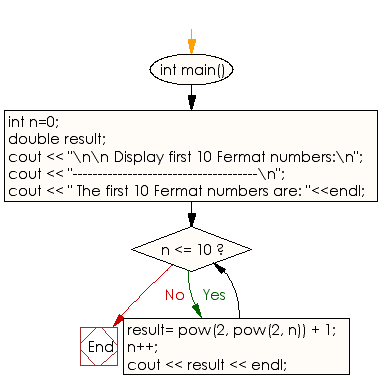﻿ C++ Exercises: Display first 10 Fermat numbers - w3resource

# C++ Exercises: Display first 10 Fermat numbers

## C++ Numbers: Exercise-33 with Solution

Write a C++ program to display the first 10 Fermat numbers.

Sample Solution:

C++ Code :

``````# include <iostream>
# include <math.h>
using namespace std;
int main()
{
int n=0;
double result;
cout << "\n\n Display first 10 Fermat numbers:\n";
cout << "-------------------------------------\n";
cout << " The first 10 Fermat numbers are: "<<endl;
while (n <= 10)
{
result= pow(2, pow(2, n)) + 1;
n++;
cout << result << endl;
}
}
``````

Sample Output:

``` Display first 10 Fermat numbers:
-------------------------------------
The first 10 Fermat numbers are:
3
5
17
257
65537
4.29497e+09
1.84467e+19
3.40282e+38
1.15792e+77
1.34078e+154
inf
```

Flowchart:C++ Code Editor: